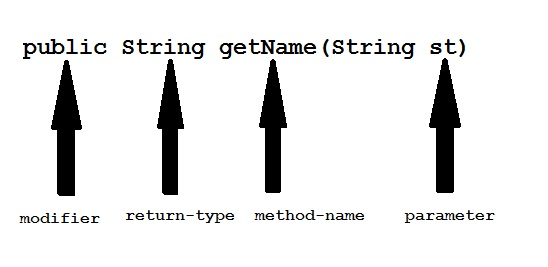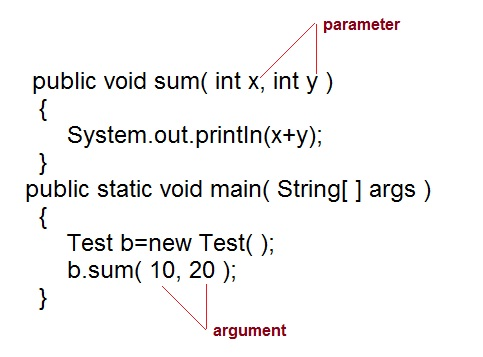Core JAVA

### Methods in Java

Method describe behavior of an object. A method is a collection of statements that are group together to perform an operation.

Syntax :

```return-type methodName(parameter-list)
{
//body of method
}
```

#### Example of a Method

```public String getName(String st)
{
String name="scanftree";
name=name+st;
return name;
}
```Modifier : Modifier are access type of method. We will discuss it in detail later.

Return Type : A method may return value. Data type of value return by a method is declare in method heading.

Method name : Actual name of the method.

Parameter : Value passed to a method.

Method body : collection of statement that defines what method does.

#### Parameter Vs. Argument

While talking about method, it is important to know the difference between two terms parameter and argument.

Parameter is variable defined by a method that receives value when the method is called. Parameter are always local to the method they dont have scope outside the method. While argument is a value that is passed to a method when it is called.#### `call-by-value` and `call-by-reference`

There are two ways to pass an argument to a method

1. call-by-value : In this approach copy of an argument value is pass to a method. Changes made to the argument value inside the method will have no effect on the arguments.
2. call-by-reference : In this reference of an argument is pass to a method. Any changes made inside the method will affect the agrument value.

NOTE : In Java, when you pass a primitive type to a method it is passed by value whereas when you pass an object of any type to a method it is passed as reference.

#### Example of `call-by-value`

```public class Test
{
public void callByValue(int x)
{
x=100;
}
public static void main(String[] args)
{
int x=50;
Test t = new Test();
t.callByValue(x);	//function call
System.out.println(x);
}

}
```

Output :

`50`

#### Example of `call-by-reference`

```public class Test
{
int x=10;
int	y=20;
public void callByReference(Test t)
{
t.x=100;
t.y=50;
}
public static void main(String[] args)
{

Test ts = new Test();
System.out.println("Before "+ts.x+" "+ts.y);
ts.callByReference(ts);
System.out.println("After "+ts.x+" "+ts.y);
}

}
```

Output :

```Before 10 20
After 100 50
```

If two or more method in a class have same name but different parameters, it is known as method overloading.

Method overloading is one of the ways through which java supports polymorphism. Method overloading can be done by changing number of arguments or by changing the data type of arguments. If two or more method have same name and same parameter list but differs in return type are not said to be overloaded method

Example :

```class Calculate
{
void sum (int a, int b)
{
System.out.println("sum is"+(a+b)) ;
}
void sum (float a, float b)
{
System.out.println("sum is"+(a+b));
}
Public static void main (String[] args)
{
Calculate  cal = new Calculate();
cal.sum (8,5);      //sum(int a, int b) is method is called.
cal.sum (4.6f, 3.8f); //sum(float a, float b) is called.
}
}```

Output :

```Sum is 13
Sum is 8.4
```

You can see that sum() method is overloaded two times. The first takes two integer arguments, the second takes two float arguments.

Example :

```class Area
{
void find(int l, int b)
{
System.out.println("Area is"+(l*b)) ;
}
void find(int l, int b,int h)
{
System.out.println("Area is"+(l*b*h));
}
public static void main (String[] args)
{
Area  ar = new Area();
ar.find(8,5);     //find(int l, int b) is method is called.
ar.find(4,6,2);    //find(int l, int b,int h) is called.
}
}```

Output :

```Area is 40
Area is 48
```

In this example the find() method is overloaded twice. The first takes two arguments to calculate area, and the second takes three arguments to calculate area.

When an overloaded method is called java look for match between the arguments to call the method and the method's parameters. This match need not always be exact, sometime when exact match is not found, Java automatic type conversion plays a vital role.

```class Area
{
void find(long l,long b)
{
System.out.println("Area is"+(l*b)) ;
}
void find(int l, int b,int h)
{
System.out.println("Area is"+(l*b*h));
}
public static void main (String[] args)
{
Area  ar = new Area();
ar.find(8,5);     //automatic type conversion from find(int,int) to find(long,long) .
ar.find(2,4,6)    //find(int l, int b,int h) is called.
}
}```

Output :

```Area is 40
Area is 48
```